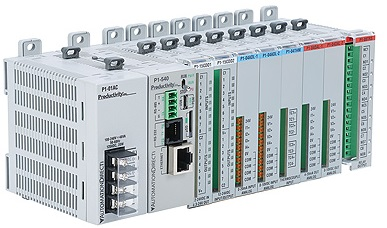# Math Instructions for PLC## Introduction

The majorities of the instructions take two input values, perform the specified arithmetic function, and output the result to an assigned memory location. For example, both the ADD and SUB instructions take a pair of input values, add or subtract them, and place the result in the specified destination. If the result of the operation exceeds the allowable value, an overflow or underflow bit is set. To learn more about the math instructions, we suggest that you read the Math Instructions Overview that follows.Use the ADD instruction to add one value (source A) to another value (source B) and place the result in the destination.

### Subtract (SUB)Use the SUB instruction to subtract one value (source B) from another (source A) and place the result in the destination.

### Multiply (MUL)Use the MUL instruction to multiply one value (source A) by another (source B) and place the result in the destination.

### Divide (DIV)Use the DIV instruction to divide one value (source A) by another (source B). The rounded quotient is then placed in the destination. If the remainder is 0.5 or greater, round up occurs in the destination. The unrounded quotient is stored in the most significant word of the math register. The remainder is placed in the least significant word of the math register.

### Clear (CLR)Use the CLR instruction to set the destination value of a word to zero.

### Square Root (SQR)When this instruction is evaluated as true, the square root of the absolute value of the source is calculated and the rounded result is placed in the destination. The instruction calculates the square root of a negative number without overflow or faults. In applications where the source value may be negative, use a comparison instruction to evaluate the source value to determine if the destination may be invalid.

### Scale with Parameters (SCP)Use the SCP instruction to produce a scaled output value that has a linear relationship between the input and scaled values. This instruction supports integer and floating point values. Use this instruction with SLC 5/03 (OS302), SLC 5/04 (OS401), and SLC 5/05 processors.

Use the following formula to convert analog input data to engineering units:

y = mx + b

Where:

y = scaled output

m = slope = (scaled MAX. – scaled MIN.) / (input MAX. – input MIN.)

x = input value

b = offset (y intercept) = scaled MIN – (input MIN. x m)

### Ramp Instruction (RMP)The Ramp (RMP) instruction provides the ability to create linear, acceleration, deceleration, and “S” curve ramp output data wave forms. This instruction provides a means to ramp analog outputs when using them to control devices such as valves.

### Swap (SWP)Use this instruction to swap the low and high bytes of a specified number of words in a bit, integer, ASCII, or string file.

### Arc Sine (ASN)Use the ASN instruction to take the arc sine of a number and store the result (in radians) in the destination. The source must be greater than or equal to -1 and less than or equal to 1. The resulting value in the destination is always greater than or equal to -Pi/2 and less than or equal to Pi/2, where Pi = 3.141592

### Natural Log (LN)Use the LN instruction to take the natural log of the value in the source and store the result in the destination. The source must be greater than zero. The resulting value in the destination is always greater than or equal to -87.33654 and less than or equal to 88.72284..